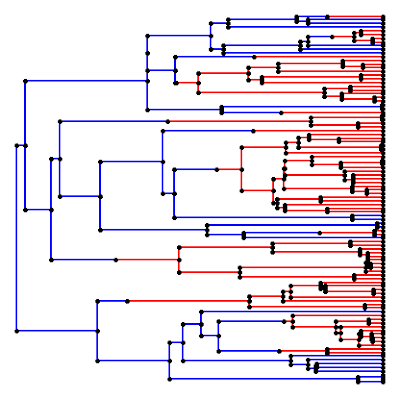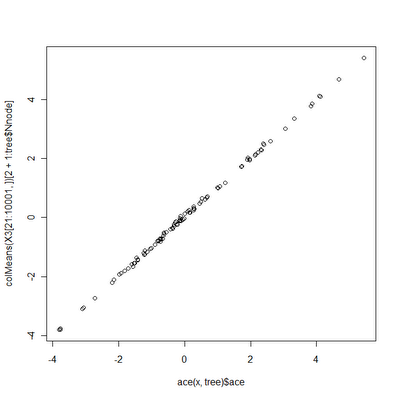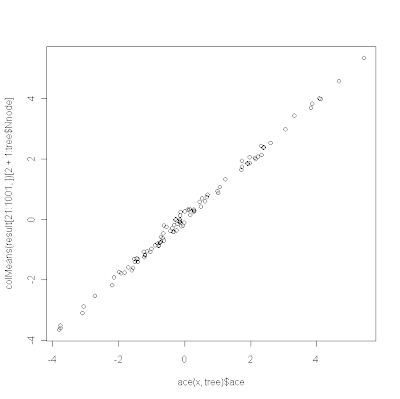## Sunday, November 27, 2011

### Simulating an irreversible discrete trait using sim.history()

A user asked how one should go about simulating a character that evolves from state "0" (say) to state "1", but not the reverse. This corresponds to the following transition matrix:

> Q
0 1
0 -1 0
1  1 0

in which we can substitute any instantaneous transition rate for the 1.0 and -1.0. Unfortunately, for various reasons sim.history doesn't work for transition rates of exactly zero - however it works fine if we substitute a value slightly greater than zero. To see this work, try the following example code that uses "geiger" and "phytools":

require(phytools)
require(geiger)
tree<-drop.tip(birthdeath.tree(b=1,d=0,taxa.stop=101),"101")
tree<-rescaleTree(tree,1)
Q<-matrix(c(-1,1,1e-10,-1e-10),2,2)
rownames(Q)<-colnames(Q)<-c("0","1")
mtree<-sim.history(tree,Q,"0")
cols<-c("blue","red"); names(cols)<-c(0,1)
plotSimmap(mtree,cols,ftype="off")

which should produce something that looks like this:Don't forget, of course, that the tip states are stored in mtree\$states, i.e.:

> mtree\$states
53  98  99  24  25  26  18  13  30  31  56  80
"0" "0" "0" "0" "0" "0" "0" "0" "1" "1" "1" "1"
81  77  ...
"1" "1"  ...

## Saturday, November 26, 2011

### phytools version 0.1-2

I just posted a new version of phytools, version 0.1-2, to my webpage, here. I have also submitted this version to CRAN (but it will probably take a day or two to be accepted, and then a few days more to percolate through all the mirror repositories). In the meantime, it can be installed in Windows by downloading the file phytools_0.1-2.zip and then typing:

> install.packages("phytools_0.1-2.zip",repos=NULL)

at the prompt. (For instructions on how to install on Mac/Linux/Unix from source, please see my website.)

New features in this version of phytools include:

1) A new function, anc.Bayes(), for Bayesian ancestral state estimation.

2) A new function, vcvPhylo(), to compute the so-called phylogenetic variance covariance matrix including ancestral nodes. I posted about this function here.

Check it out!

## Friday, November 25, 2011

### Major speed up of anc.Bayes()

I just realized how to score a major speed-up in computation time from my new Bayesian ancestral character estimation function for continuous traits (anc.Bayes). Last night I documented what I thought at the time was a significant speed improvement. By pulling inversion of the phylogenetic VCV matrix out of the likelihood function (meaning that we only have to do this once instead of every generation of the MCMC), I was able to cut computation time in half (from about 60s on a 100 taxon tree to about 30s). What I didn't realize at the time was that I could also pull out calculation of the determinant of the matrix. The determinant we want is log(|σ2C|); the way we avoid recalculating this every generation of the MCMC (in which σ2 can potentially differ) is by taking advantage of the equality:

log(|σ2C|) = nlog(σ2) + log(|C|)

I actually described this in an earlier paper with Luke Harmon (Revell & Harmon 2008, p. 318), but did not think to apply it to this problem until now. [Note that there is actually a small error in equation (5) of our article: n·r·log(k) should be n·r·log(k)/2.]

This version runs much faster: now about 4s for the same dataset as before. Unbelievable! Anyway, I have posted the new version on my website here.

This version is so much faster that I can easily run it for a million generations on the 100 species tree from before. If I plot the estimates from the Bayesian MCMC run with an uninformative prior against the values from ML estimation using ace() in the ape package, we can see that they are even more tightly correlated than before:

> source("anc.Bayes.R")
> source("vcvPhylo.R")
> X<-anc.Bayes(tree,x,ngen=1000000)
> plot(ace(x,tree)\$ace,colMeans(X[21:10001,])[2+1:tree\$Nnode])(compare to here). The above code took about 6 minutes to run, whereas the first version of anc.Bayes would've needed about 100 minutes for the same calculations.

I haven't mentioned MCMC diagnostics, priors, or the utility of Bayesian inference at all yet. Hopefully, these will be addressed in future posts.

### Improvement to anc.Bayes()

I have already identified a major area in which I can easily improve the computation time of anc.Bayes(), my new function for Bayesian MCMC ancestral state estimation. Specifically, after having thought myself quite clever for using dmnorm() in the mnormt library (see post here), I realized that this was actually extremely computationally inefficient - especially for large matrices. This is because by using dmnorm() I'm essentially forced R to invert our VCV matrix (which is of order number of tips + number of nodes) every generation of the MCMC. This is totally unnecessary and I can easily create a custom function to compute the MVN probability density in which I avoid inverting the phylogenetic VCV matrix on each call. This function is below (it still requires that we invert C once), and I will post a new version of anc.Bayes() tomorrow.

likelihood<-function(C,invC,x,sig2,a,y){
logLik<--(c(x,y)-a)%*%invC%*%(c(x,y)-a)/(2*sig2) -nrow(invC)*log(2*pi)/2 -determinant(sig2*C,log=T)\$modulus/2
return(logLik)
}

## Thursday, November 24, 2011

### Bayesian ancestral character estimation

I've just been working on a new function for Bayesian ancestral character estimation (anc.Bayes). I'm still working out some of the bugs, but I have nonetheless posted a preliminary version online, here. (It also uses vcvPhylo(), which can be downloaded here).

1) If we take advantage of the multivariate normal density function dmnorm in the mnormt package, and my function vcvPhylo(), computing the likelihood (which we will use to calculate the posterior odds ratio) can be done in just a few lines of code:

C<-vcvPhylo(tree)
# function returns the log-likelihood
likelihood<-function(C,x,sig2,a,y){
logLik<-dmnorm(x=c(x,y),mean=rep(a,nrow(C)),varcov=sig2*C, log=TRUE)
return(logLik)
}

in which x is the data for species, y are the ancestral character values, a is the state at the root node, and sig2 is the evolutionary rate.

2. All the control parameters of the Bayesian MCMC (starting values, proposal distributions, and prior probabilities) are accessible to the user - but I have also tried to give the function the capacity to give reasonable values for these things if they are not provided by the user.

Although I'll warn again that this I am still working on this, it is not too soon to try it out. Let's simulate, run the MCMC, and then compare the results to ML estimates obtained using ace() in the ape package:

> require(phytools)
> source("vcvPhylo/vcvPhylo.R")
> source("anc.Bayes/anc.Bayes.R")
> set.seed(100)
> tree<-rtree(100)
> x<-fastBM(tree,sig2=2)
> result<-anc.Bayes(tree,x,ngen=100000)
Control parameters (set by user or default):
List of 7
\$ sig2   : num 2.03
\$ a      : num [1, 1] 0.00737
\$ y      : num [1:98] 0.00737 0.00737 0.00737 0.00737 0.00737 ...
\$ pr.mean: num [1:100] 1000 0 0 0 0 0 0 0 0 0 ...
\$ pr.var : num [1:100] 1e+06 1e+03 1e+03 1e+03 1e+03 1e+03 1e+03 1e+03 1e+03 1e+03 ...
\$ prop   : num [1:100] 0.142 0.142 0.142 0.142 0.142 ...
\$ sample : num 100
Starting MCMC...
Done MCMC.
> # plot the result against the MLEs
> plot(ace(x,tree)\$ace,colMeans(result[21:1001,])[2+1:tree\$Nnode])

Here, we get the following result:Obviously, I will post more on this as I improve it and I will also try to provide more guidance on MCMC control and priors.

## Wednesday, November 23, 2011

### Faster vcvPhylo() with ancestral nodes

In my last post I put up a simple version vcv.phylo() (which returns a matrix containing the height above the root of each pair of species in the tree, sometimes called the phylogenetic variance-covariance matrix), but that included ancestral nodes. I pulled this out of an old function of mine, anc.trend(), so I figured I could do better - and after some tinkering I came up with a much improved version, as follows:

vcvPhylo2<-function(tree,anc.nodes=T){
n<-length(tree\$tip.label)
h<-nodeHeights(tree)[order(tree\$edge[,2]),2]
h<-c(h[1:n],0,h[(n+1):length(h)])
M<-mrca(tree,full=anc.nodes)[c(1:n,anc.nodes*(n+2:tree\$Nnode)),c(1:n,anc.nodes*(n+2:tree\$Nnode))]
C<-matrix(h[M],nrow(M),ncol(M))
if(anc.nodes) rownames(C)<-colnames(C)<-c(tree\$tip.label,n+2:tree\$Nnode)
else rownames(C)<-colnames(C)<-tree\$tip.label
return(C)
}

A couple of tricks in here:

1) Rather than calling dist.nodes(), this function uses the phytools function nodeHeights, which returns a matrix of the dimensions of tree\$edge, containing the height above the root of every node, and is quite fast.

2) The function then uses mrca(...,full=T) in the ape package, which returns the MRCA of every pair of species and nodes. This essentially serves as an index to construct our VCV matrix from the node heights!

All of this is done with the following lines of code:

# this gets a vector of height by node number
h<-nodeHeights(tree)[order(tree\$edge[,2]),2]
# this inserts a height of zero for the root node (node n+1 for n species)
h<-c(h[1:n],0,h[(n+1):length(h)])
# this is our index matrix
M<-mrca(tree,full=T)
# this is our VCV matrix
C<-matrix(h[M],nrow(M),ncol(M))

Let's compare computation time to vcv.phylo and our earlier version (vcvPhylo1):

> tree<-rtree(500)
> system.time(X<-vcv.phylo(tree)) # only tips
user  system elapsed
2.20    0.00    2.22
> system.time(Y<-vcvPhylo1(tree)) # tips & nodes, old version
user  system elapsed
7.68    0.03    7.73
> system.time(Z<-vcvPhylo2(tree)) # tips & nodes, new version
user  system elapsed
3.49    0.05    3.54
> all(Y==Z)
 TRUE
> all(X==Z[c(1:500),c(1:500)])
 TRUE

Cool! BTW - Happy Thanksgiving to all the blog readers.

## Monday, November 21, 2011

### vcv.phylo() with ancestral nodes

An R-sig-phylo user just posted a request for a function to compute the so-called phylogenetic variance-covariance matrix. That is, the N×N matrix (for N species) containing in position i,j the height above the root of the MRCA of species i and j (as done in the ape function vcv.phylo) - but including ancestral nodes. As it turns out, I have a code fragment to do this in my function anc.trend() (link here). I pasted the fragment into a function vcvPhylo() as follows:

vcvPhylo<-function(phy,anc.nodes=T){
if(anc.nodes){
D<-dist.nodes(phy)
ntips<-length(phy\$tip.label)
Cii<-D[ntips+1,]
C<-D; C[,]<-0
for(i in 1:nrow(D)) for(j in 1:ncol(D))
C[i,j]<-(Cii[i]+Cii[j]-D[i,j])/2
dimnames(C)[][1:length(phy\$tip)]<-dimnames(C)[][1:length(phy\$tip)]<-phy\$tip.label
C<-C[c(1:ntips,(ntips+2):nrow(C)),c(1:ntips,(ntips+2):ncol(C))]
return(C)
} else return(vcv.phylo(phy))
}

and it seems to do the trick.

## Thursday, November 17, 2011

### phytools version 0.1-1

I just released the latest version of phytools to my website, here. The new features and functions of this version include:

1) A new function, make.era.map(), which allows the user to map temporal eras on the tree. More details in a prior post.

2) A user requested update to phyl.RMA() allowing for a fixed value of the λ parameter. More on this here.

3) A new function, brownieREML, which does much faster REML model fitting of the multiple rate Brownian evolution model of O'Meara et al. (2006). More on this here.

4) A couple of updates to phylosig() that allow the incorporation of sampling error into estimates of phylogenetic signal using K & λ. The method for including sampling error is based on Ives et al. (2007).

I also added a CITATION file. The CITATION file tells R how to respond to the function call:

> citation("phytools").

My CITATION file looks something like the following:

citHeader("To cite phytools in publication use:")
citEntry(
entry="Manual",
title="phytools: An R package for phylogenetic comparative
biology (and other things).",
author="Liam J. Revell",
year=2012,
url="http://cran.r-project.org/web/packages/phytools/",
textVersion=paste("Revell, L. J. (In press)","phytools: An R package for phylogenetic comparative biology (and other things)","Methods Ecol. Evol.")
)
citFooter("As phytools is continually evolving, you may want to cite its version number. Find it with 'help(package=phytools)'.")

and calls to citation("phytools") will now return the following:

> citation("phytools")

To cite phytools in publication use:

Revell, L. J. (In press) phytools: An R package for phylogenetic
comparative biology (and other things) Methods Ecol. Evol.

A BibTeX entry for LaTeX users is

@Manual{,
title = {phytools: An R package for phylogenetic comparative
biology (and other things).},
author = {Liam J. Revell},
year = {2012},
url = {http://cran.r-project.org/web/packages/phytools/},
}

As phytools is continually evolving, you may want to cite its version number. Find it with 'help(package=phytools)'.

Cool.

## Wednesday, November 16, 2011

### Including "measurement error" in the estimation of λ

I just posted a new version of the function phylosig() which now allows the user to incorporate measurement error in the estimation of Pagel's λ. Pagel's λ is usually estimated using maximum likelihood, so it was straightforward to condition the estimation of λ & σ2 (the rate of evolution) on an observed set of standard errors. The code for this new version of the function is here. Unfortunately, as I have added capabilities to this function, I have done so in a haphazard fashion, which has resulted in code for this function that has become a little bit more expansive than necessary (compare, for instance, to the code for my new REML version of brownie.lite, here). At some point I will try and clean this up.

To add measurement error to the estimation of λ is not too difficult. Basically, under the λ model we expect that the multivariate distribution of phenotypic values among species will be given by σ2Cλ; where Cλ is an n×n matrix (for n species) containing, in the diagonal, the height of each species above the root; and, in each off-diagonal element Cλ(i,j), the height above the root node of the MRCA of species i and species j multiplied by the coefficient λ. In other words: x ~ MVN(σ2Cλ). Given a set of values for x and a tree, we can estimate λ using likelihood.

To incorporate measurement error (that is, estimation error for the species means for the trait of interest), we just have x ~ MVN(σ2Cλ + E), where E is a matrix containing the error variance and covariances among species. If we assume that estimation error is uncorrelated among species, then E is just a diagonal matrix containing the square of the estimation error for each species. (The assumption that measurement error is independent among species is reasonably safe - but it might be false if, for instance, samples were measured by different investigators or in different years; e.g., see Ives et al. 2007).

The way to run phylosig() with measurement error is simply by supplying the additional vector, se, containing the standard errors for each species. names(se) should contain the species names (corresponding to tree\$tip.label, although they need not be in the same order).

To see how the function works, let's try the following example:

> require(phytools); require(geiger)
> set.seed(2)
> gen.lambda<-0.7
> tree<-drop.tip(birthdeath.tree(b=1,d=0,taxa.stop=501),"501")
> se<-sqrt(rchisq(n=500,df=2)); names(se)<-tree\$tip.label
> x<-fastBM(lambdaTree(tree,gen.lambda),sig2=1)
> e<-rnorm(n=500,sd=se)
> xe<-x+e
> phylosig(tree,x,method="lambda")
\$lambda
 0.7141959
\$logL
 -1018.6
> phylosig(tree,xe,method="lambda")
\$lambda
 0.5431885
\$logL
 -1153.855
> phylosig(tree,xe,method="lambda",se=se)
\$lambda
 0.7274975
\$sig2
 1.161162
\$logL
 -1135.698

1) The decrease in estimated phylogenetic signal when measurement error is ignored is exactly what we expect for the λ model. This is because measurement error and the λ model have exactly the same effect on the distribution of variation among species (that is, both add extra variance to the tips).

2) The likelihoods for the first result cannot be compared to the likelihoods for the second or third result because it has been obtained for different data (in this case, the hypothetical data for species means without error - the data we never actually have in empirical studies). The likelihoods for results 2 & 3 can be compared because they are obtained for the same data. In this case, the likelihood improved dramatically for the model with measurement error. This suggests that the tree & measurement errors are much better at explaining the observed data than is the tree alone, under the λ model.

## Tuesday, November 15, 2011

### REML version of brownie.lite

I just posted a new, simplified REML version of brownie.lite(). brownie.lite() is based on the method of O'Meara et al. (2006) in which we fit different evolutionary rates to different, pre-defined parts of a phylogenetic tree with branch lengths.

The REML version works by using a likelihood function for the phylogenetically independent contrasts rather than for the original data. We optimize the multi-rate model, in this case, by optimization the (restricted) likelihood of different rescaling of the different branch lengths of the tree. The problem with maximizing the likelihood for the original data is that it involves constructing and inverting matrices of dimension n×n for n species. By maximizing a likelihood function for the contrasts, we can avoid this very computationally intensive operation. In addition, REML (unlike ML) produces unbiased parameter estimates. ML estimates are biased downward by a factor of n/(n-1).

To see the improvement in computation time that is provided by REML in this case, download the source file (here), and try out the following exercise. (Note that on a tree with 1000 terminal species, as in this example, be forewarned that brownie.lite() will take several minutes to run.)

> require(geiger); require(phytools)
> set.seed(10) # for repeatability
> tree<-drop.tip(birthdeath.tree(b=1,d=0,taxa.stop=1001),"1001")
> tree<-rescaleTree(tree,1)
> tree<-sim.history(tree,matrix(c(-1,1,1,-1),2,2))
> x<-sim.rates(tree,c(1,10))
names absent from sig2: assuming same order as \$mapped.edge
> system.time(result1<-brownie.lite(tree,x))
user  system elapsed
353.53    3.39  357.70
> source("brownieREML.R")
> system.time(result2<-brownieREML(tree,x))
user  system elapsed
0.28    0.00    0.28
> result1
\$sig2.single
 6.477488
...
\$logL1
 -1348.224
...
\$sig2.multiple
1        2
1.010363 9.819315
...
\$logL.multiple
 -1160.006
...
> result2
\$sig2.single
 6.483972
\$logL1
 -1347.577
\$sig2.multiple
1        2
1.012534 9.824353
\$logL2
 -1159.737
\$convergence
 TRUE

I anticipate that brownieREML() will be added to future versions of the "phytools" library.

## Friday, November 11, 2011

### phytools manuscript accepted

Quick post - the manuscript describing "phytools" was recently accepted at Methods in Ecology & Evolution. See blog post here.

### New version of phyl.RMA() with fixed lambda

I just posted a new version of my R function for phylogenetic reduced major axis regression (RMA), called phyl.RMA(). Since the RMA slope is merely computed as the variance ratio, this function just computes phylogenetic variances and then takes their ratio. The function also (for method="lambda") first performs joint estimation of Pagel's λ (e.g., see Freckleton et al. 2002). Direct link to the code is here.

The new feature of this version of the function (as requested by a user) is merely that the value of λ can be fixed by the user rather than estimated. This is done by calling phyl.RMA(...,fixed=TRUE,lambda=XX). So, for instance, try the following:

> require(geiger)
> require(phytools)
> source("phyl.RMA.R")
> tree<-drop.tip(birthdeath.tree(b=1,d=0,taxa.stop=201),"201")
> X<-fastBM(lambdaTree(tree,0.7),nsim=2)
> phyl.RMA(X[,1],X[,2],tree,method="lambda")
\$RMA.beta
 -0.0866432 1.0590274
\$V
x y
x 0.97406967 -0.03095092
y -0.03095092 1.09245711
\$lambda
 0.734092
\$logL
 -770.6879
\$resid
[,1]
21 -5.863653843
101 -5.841695560
...

If we wanted to know, say, if the estimated value of λ (here ~0.73) was significantly different than 0.0 (or any other value), we can fix λ at 0.0 and recalculate:

> phyl.RMA(X[,1],X[,2],tree,method="lambda",fixed=T,lambda=0)
\$RMA.beta
 -0.7000868 0.9720490

\$V
x y
x 0.75527418 -0.09025328
y -0.09025328 0.71364296

\$lambda
 0

\$logL
 -845.7699

\$resid
[,1]
21 -5.01116336
101 -4.87370275
...

Cool!

Unfortunately, it has just been reported to me that we have had an escaped anole from the common garden Anolis carolinensis rearing experiment that is presently taking place in my lab. Time to go lizard hunting!